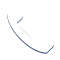# INDEX + MATCH: An Alternative to VLOOKUP

Have you ever heard of INDEX + MATCH? VLOOKUP is a handy shortcut that helps you use Microsoft Excel more easily. While many users love the VLOOKUP function, it does have some limitations. Luckily, there is an alternative to VLOOKUP that you might not know about: INDEX + MATCH. In this article, we’ll tell you how to use this little-known function to make Excel even easier to use.## VLOOKUP

VLOOKUP, short for “Vertical Lookup,” is a built-in Excel function that works with data that is organized into columns. For a specified value, VLOOKUP finds (or looks up) the value in one column of data, and returns the corresponding value in another column.

### Limitations of VLOOKUP

However, VLOOKUP does have limitations. For example, it only reads from left to right. So, if you need to look up a value to the left of a reference column, you will need to rearrange your worksheet in order to use it.

VLOOKUP also canvasses many columns, when in reality you are only using two. The standard syntax only references relative column values. If you modify the table, you will need to do more reorganization and function rewriting, which is tedious work. Plus, it can create issues in copying the function to other columns.

## INDEX + MATCH

Luckily, there is an alternative to VLOOKUP, and it is INDEX + MATCH.

INDEX + MATCH has the same functionality of VLOOKUP, plus a few additional benefits.

Next, we’ll teach you how to use INDEX + MATCH.## How to Use INDEX MATCH

One of the most overlooked parts of Excel is that you can use various functions together in order to create a more powerful function, like INDEX + MATCH.

### INDEX

=INDEX(array, row number)

The INDEX function takes a user array, a set of values such as a column, and returns the value of the cell at that particular position.

### MATCH

=MATCH(lookup value, lookup array, match type)

MATCH () is another simple function. It is similar to the INDEX function, however, instead of returning a value, it instead gives you a number identifying the relative position of a value within the array. MATCH requires three arguments: the value, the array to search, and the match type. The match type will typically be set to zero, but it depends on your usage. Zero signifies you want only an exact match, and 1 or -1 signifies if there is no exact match you want the next closest value.## Putting INDEX + MATCH Into Practice

With INDEX + MATCH, you can combine these two functions to perform something similar to VLOOKUP. (Just with a little more typing.) INDEX returns a specific value, which is also the primary function of VLOOKUP. Now, you need a way to find the row position. If you have to find this manually, you lose the utility of a function. When you use MATCH in the second argument, you take its output, a relative position, and push that into the INDEX function, solving that problem.

INDEX + MATCH is helpful because it involves smaller data selection. This helps you run large data sets faster, too. Plus, INDEX + MATCH can read left or right, no matter where you put the columns.

Now, start using this function and see how you like it! It’s a great function to know that can improve your lookup abilities in Microsoft Excel.

For more tips for web, like advanced tricks for how to search on Google, check out the rest of our marketing blog!

## FAQs:

• What is VLOOKUP in Excel?
• What are the limitations of VLOOKUP?
• How do I use Index Match in Excel?
• What alternative can I use to VLOOKUP?
• Why is INDEX + MATCH a good trick to know?Call Now